# 准静态条件下金属材料的临界断裂准则研究1)

Yu Simiao, Cai Lixun2), Yao Di, Bao Chen, Chen Hui, Peng Yunqiang, Han Guangzhao

Applied Mechanics and Structure Safety Key Laboratory of Sichuan Province, School of Mechanics and Engineering, Southwest Jiaotong University, Chengdu 610031, China

Abstract

For 10 types of specimens with different constraints, ductile fracture tests of 9 metal materials under unidirection loading were performed, and their load-displacement relations were measured. Based on the load-displacement curves of notched round bar, the full-range equivalent constitutive relationships of materials up to failure were obtained by FAT (finite-element-analysis aided testing) method. Further, the simulated force-displacement curves for different specimens were obtained based on the full-range constitutive relations, which agree well with the experimental force-displacement curves. The results demonstrate that the full-range constitutive relations up to failure obtained by FAT method have uniqueness for the materials. The critical fracture parameters such as critical stress, critical strain and critical stress triaxiality are investigated by failure simulations for the 36 specimens with different constraints. The first principal stress is suggested to be the master parameter to control ductile fracture. By analyzing the critical behaviors of the specimens which are smooth, notched and cracked, respectively, a unified strength criterion for ductile materials with stress triaxiality varying from $-1$ to 3 is proposed.

Keywords： ductile materials ; finite element aided testing method ; plastic deformation ; critical fracture criterion

0

Yu Simiao, Cai Lixun, Yao Di, Bao Chen, Chen Hui, Peng Yunqiang, Han Guangzhao. THE CRITICAL STRENGTH CRITERION OF METAL MATERIALS UNDER QUASI-STATIC LOADING1)[J]. Acta Mechanica Sinica, 2018, 50(5): 1063-1080 https://doi.org/10.6052/0459-1879-18-172

## 引言

Freudenthal 准则

\begin{equation*}\int^{\varepsilon_{\text {eqf}}}_0 \sigma_{\text{eq}}\text d\varepsilon_{\text{eq}}=C_{\text{cr}}\end{equation*}

Clockcroft-Latham 准则

\begin{equation*}\int^{\varepsilon_{\text {eqf}}}_0 \sigma_{\text{1}}\text d\varepsilon_{\text{eq}}=C_{\text{cr}}\end{equation*}

Rice-Tracy 准则

\begin{equation*}\int^{\varepsilon_{\text {eqf}}}_0 e^{\sigma^*}\text d\varepsilon_{\text{eq}}=C_{\text{cr}}\end{equation*}

Brozzo 准则

\begin{equation*}\int^{\varepsilon_{\text {eqf}}}_0 \dfrac{2}{3}\dfrac{\sigma_1}{\sigma_1-\sigma_\text m}\text d\varepsilon_{\text{eq}}=C_{\text{cr}}\end{equation*}

Oh 准则

\begin{equation*}\int^{\varepsilon_{\text {eqf}}}_0 \dfrac{\sigma_1}{\sigma_{\text {eq}}}\text d\varepsilon_{\text{eq}}=C_{\text{cr}}\end{equation*}

1950年, Freudenthal假定在塑性变形过程中材料单位体积内所吸收的广义塑性功达到临界值时材料发生断裂, 由此提出了Freudenthal断裂准则. Freudenthal针对延性材料断裂所提出的临界塑性功假定主导了后续几十年的断裂强度研究. 1968年, Clockcroft和Latham通过拉伸试验对Freudenthal准则进行验证, 修正提出了Clockcroft-Latham拉伸应变能准则. 1969年, Rice和Tracy在延性材料断裂准则研究中第1次提出应力三轴度概念, 提出了基于应力三轴度的Rice-Tracy准则. 1970年代, Brozzo和Oh[4-5]分别强调了静水应力和等效应力在延性断裂行为中的重要作用, 并对Clockcroft-Latham准则进行了修正, 提出了Brozzo准则和Oh准则.

1880年代以后, 随着有限元技术和试验技术的发展, 延性断裂准则的研究也有了新的变化, 主要包括以下3 个方向:

\begin{equation*}\varepsilon_{\text{eqf}}=C_1{\rm exp}(C_2\sigma^*)+C_3\tag*{(1)}\end{equation*}

\begin{equation*}\sigma_{\rm lf}'={\rm ln}(\sigma^*_\text f)+S'\tag*{(3)}\end{equation*}

## 1 研究条件

### 1.1 试验材料、设备与试样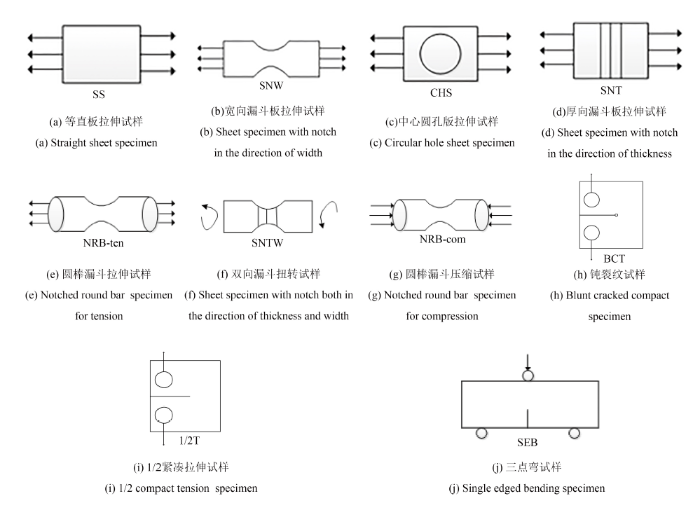Fig.1   Configuration of specimens

Table 1   Completed configuration of specimens for materials experiments

MaterialsSpecimens
T225SS SNW CHS NRB-tension SNTW NRB-compression SEB
60Si2MoSS SNW CHS NRB-tension
6061SS SNW CHS NRB-tension 1/2T
A508-3SNT SNW CHS NRB-tension BCT
980GCHS NRB-tension
316LSNT CHS NRB-tension
Q345CHS NRB-tension BCT
30CrSNW CHS NRB-tension BCT
3Cr13SNW CHS NRB-tension BCT

### 1.2 材料真应力-真应变曲线

\begin{equation*}\left.\begin{split}\sigma_{\rm Eng}=\dfrac{4F}{\textrm{π}d^2}\\\varepsilon_{\rm Eng}=\dfrac{\Delta L}{L}~~\end{split}\right\}\Rightarrow\left.\begin{split}\sigma_{\rm T}=\sigma_{\rm Eng}(1+\varepsilon_{\rm Eng}) \varepsilon_{\rm T}={\rm ln}(1+\varepsilon_{\rm Eng})\quad~\end{split}\right\}\tag*{(4)}\end{equation*}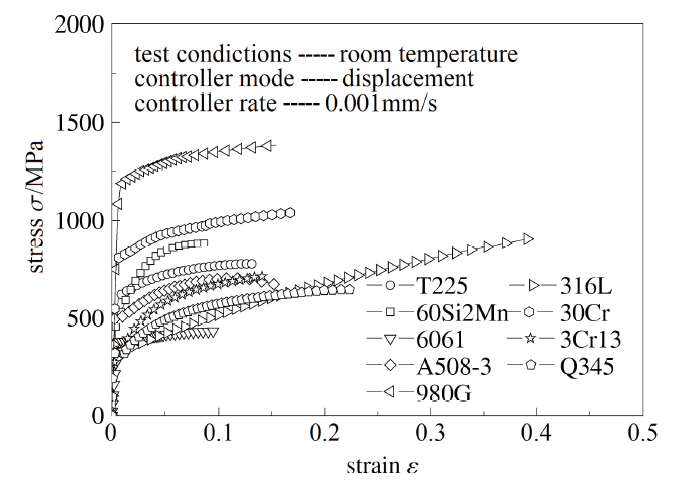Fig.2   The uniaxial true stress-true strain curves before necking

\begin{equation*}\varepsilon_\text T=\varepsilon_{\text e_\text T}+\varepsilon_{\text p_\text T}=\dfrac{\sigma_\text T}{E}+\Bigg(\dfrac{\sigma_\text T}{K}\Bigg)^N\tag*{(5)}\end{equation*}

Table 2   Parameters of mechanical properties for materials

MaterialsE/GPaKN
T22511093212.10
60Si2Mo20916404.56
60617250415.80
A508-31959327.07
980G210152019.80
316L19512902.54
Q3452108885.16
30Cr20311304.49
3Cr13204121011.90

### 1.3 有限元分析模型

Table 3   Finite element models of specimens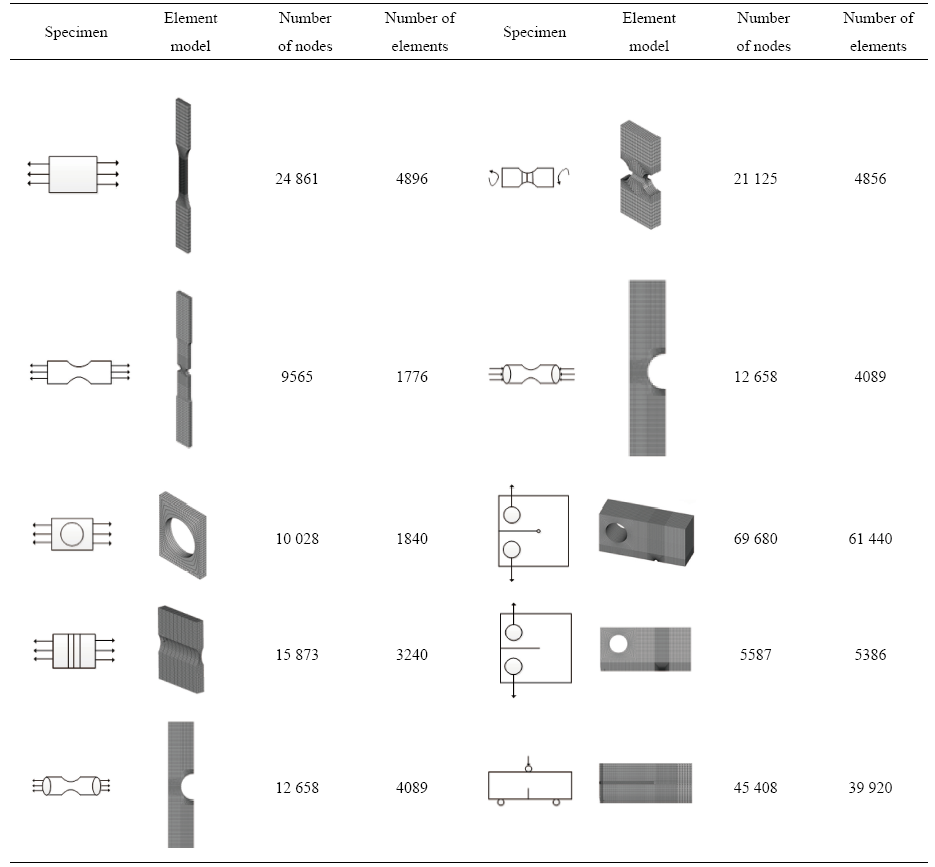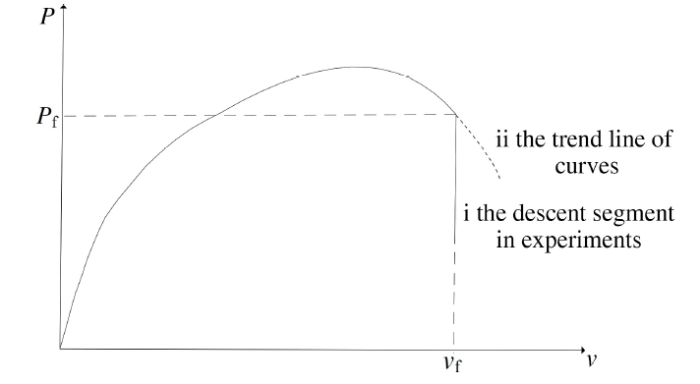Fig.3   A schematic diagram of fracture displacement

### 1.4 FAT方法

FAT方法的算法框图如图4所示, 首先完成圆棒漏斗试样的拉伸试验, 得到$P$-$v$曲线, 根据$P$-$v$曲线编写ANSYS APDL 命令流程序, 实现FAT 方法的自动运算.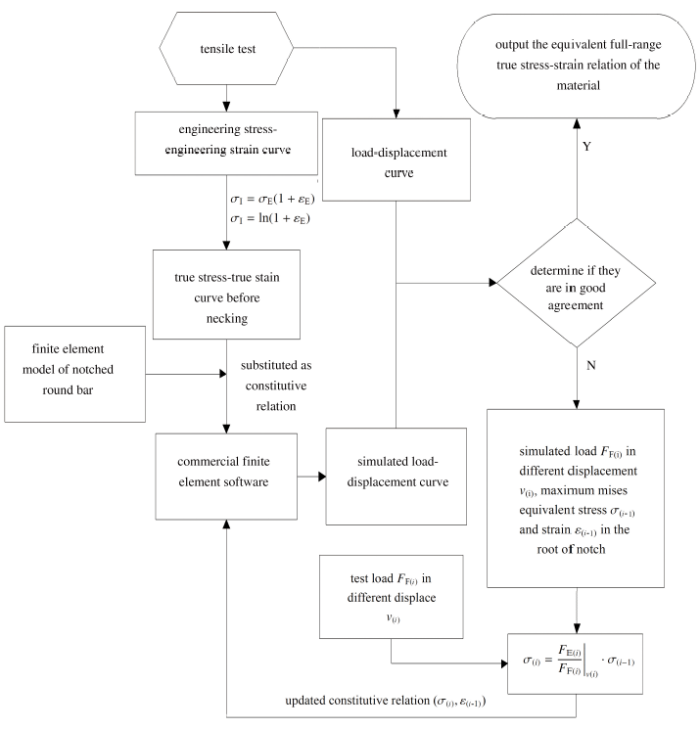Fig.4   Basic process of FAT method

## 2 FAT方法有效性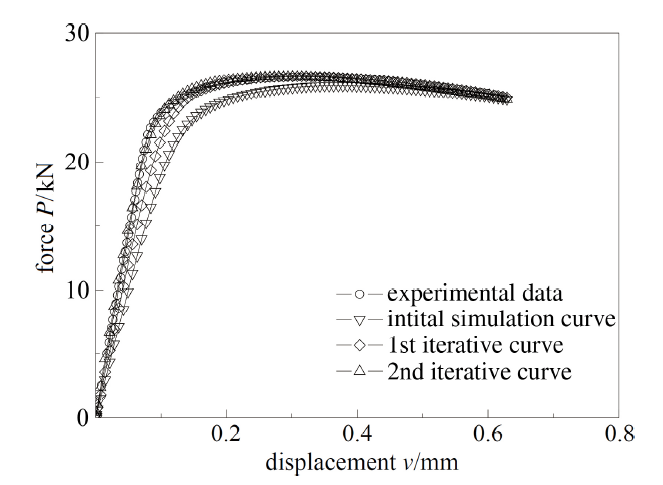Fig.5   The iterative $P$-$v$ curves of T225 alloy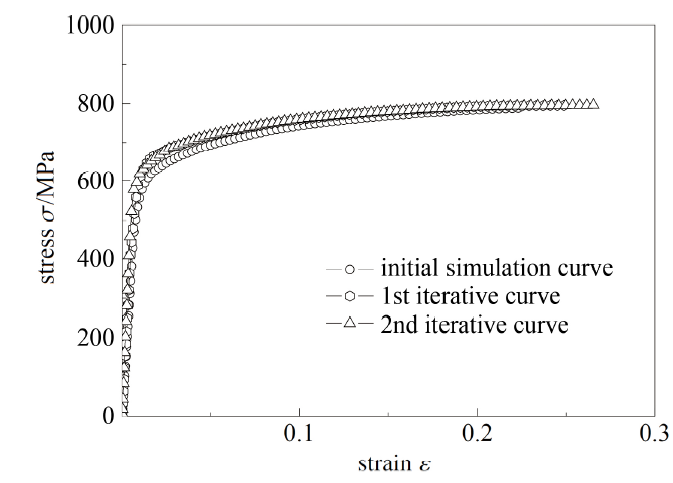Fig.6   The iterative $\sigma$-$\varepsilon$ curves of T225 alloy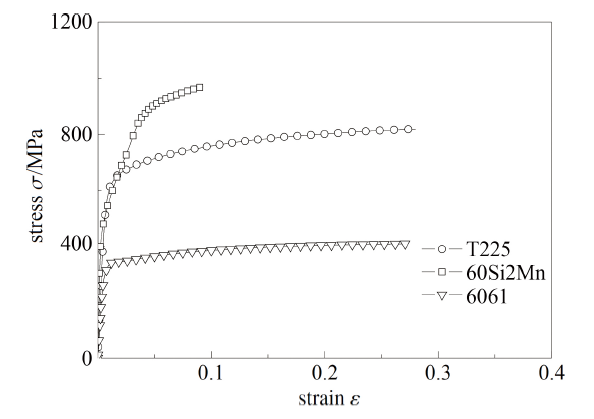Fig.7   The full-range constitutive relationships of low to medium ductile materials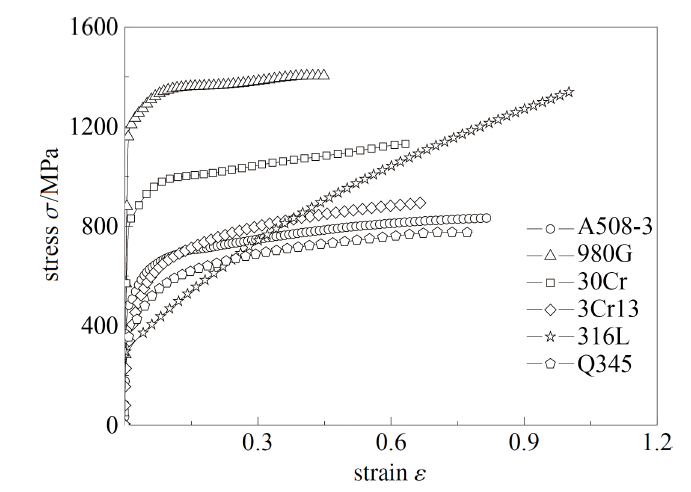Fig.8   The full-range constitutive relationships of high ductile materials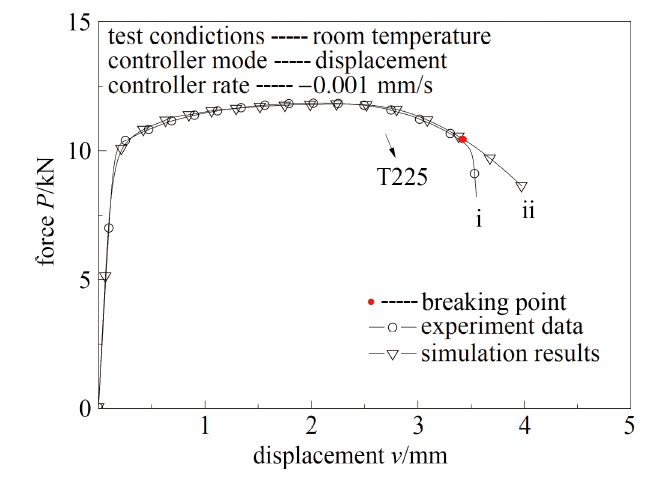Fig.9   The $P$-$v$ curves of SS specimens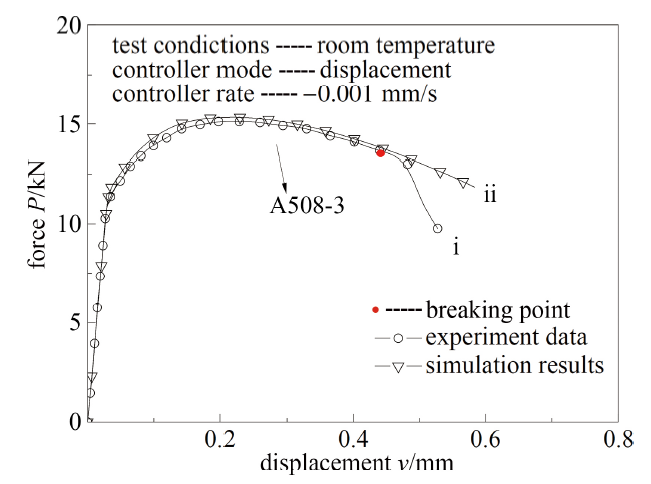Fig.10   The $P$-$v$ curves of SNT specimens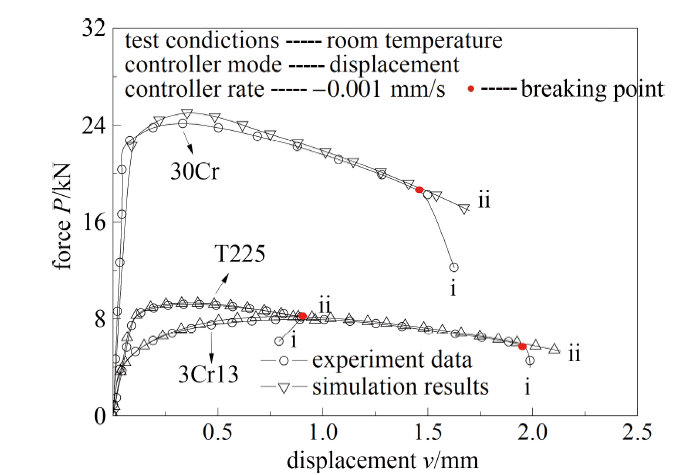Fig.11   The $P$-$v$ curves of SNW specimens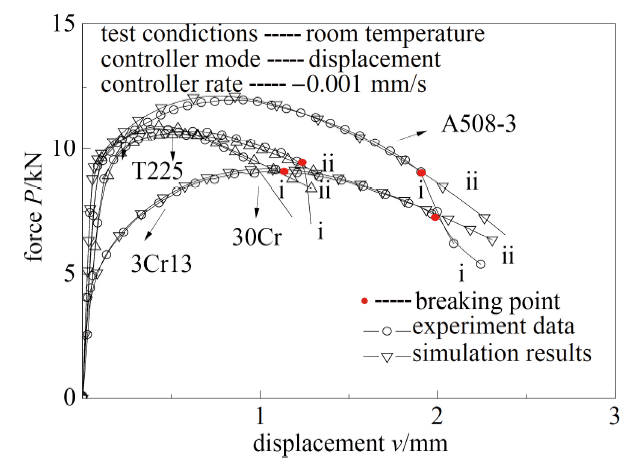Fig.12   The $P$-$v$ curves of CHS specimens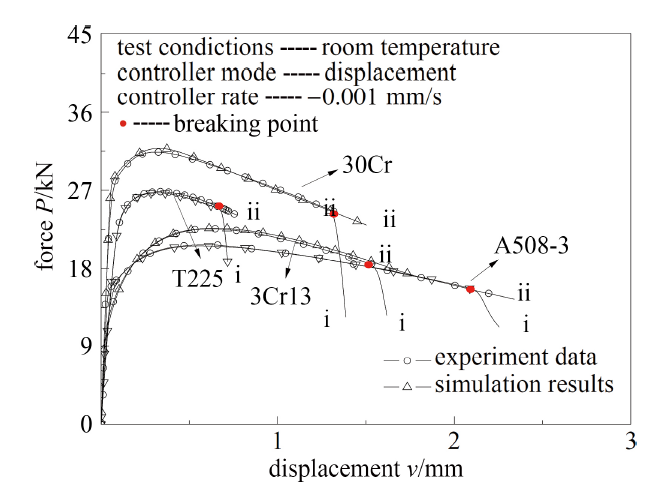Fig.13   The tensile $P$-$v$ curves of NRB specimens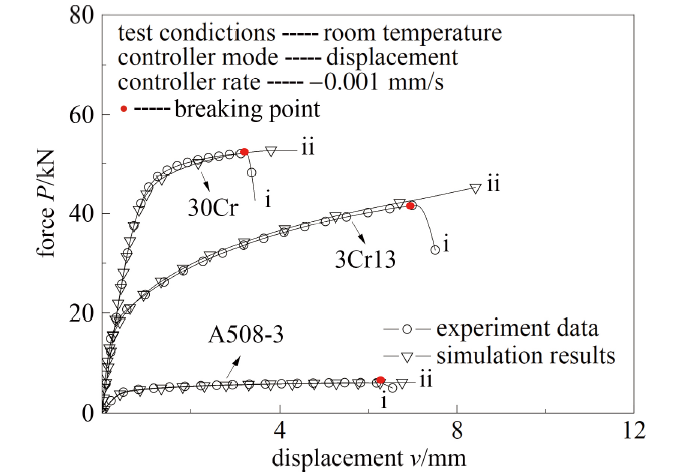Fig.14   The $P$-$v$ curves of BCT specimens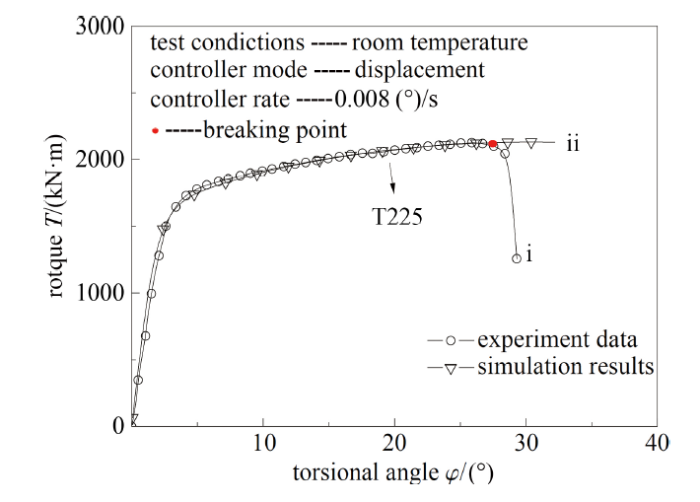Fig.15   The $T$-$A$ curves of SNTW specimens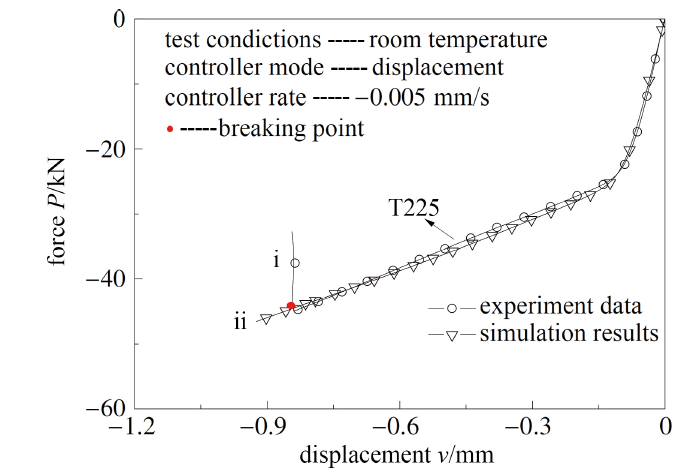Fig.16   The compressed $P$-$v$ curves of NRB specimens

FAT方法的应用广泛, 可用于获取各向同性材料直至破坏的全程本构关系曲线、断裂应力、断裂应变、应力三轴度等材料性能, 所得结果的准确性是传统单轴拉伸试验无法达到的, 更有利于大变形分析, 对工程中的结构设计和安全评估具有重要意义.

## 3 构型试样的断裂分析

### 3.1 试样的断裂面分析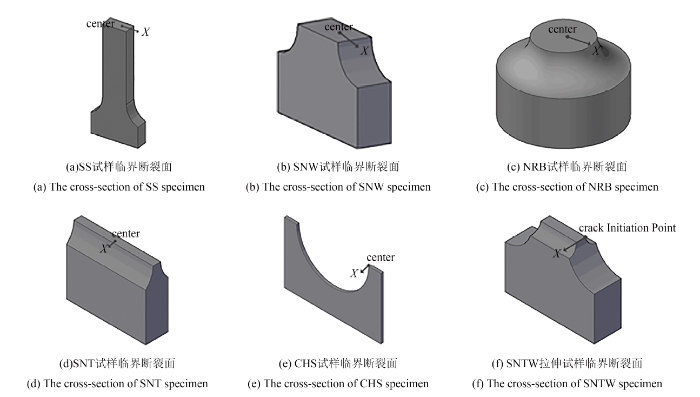Fig.17   Coordinate system on the cross-section of specimens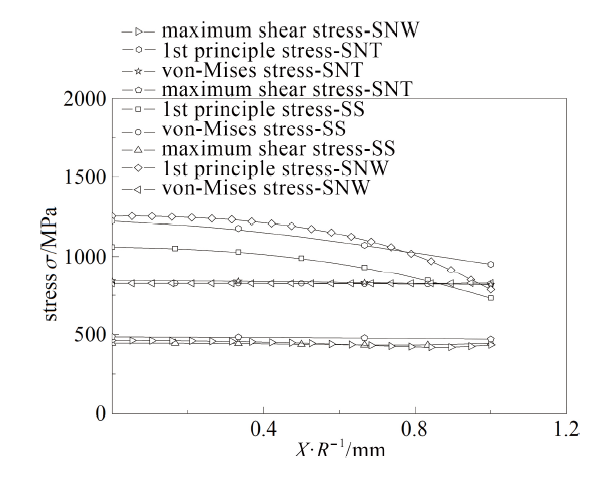Fig.18   Stress distribution on $X$ direction of tension and compression specimens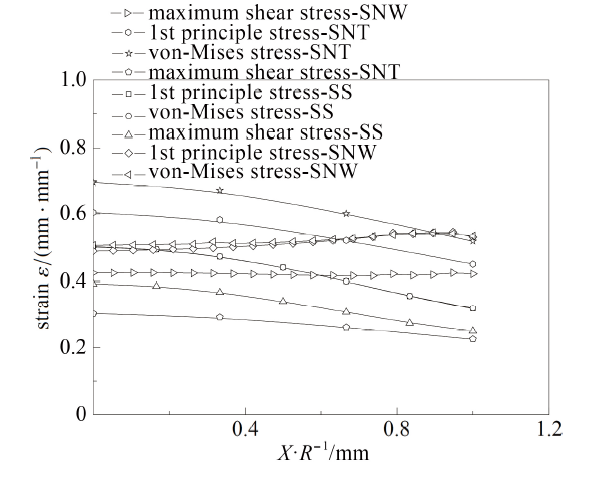Fig.19   Strain distribution on $X$ direction of SS, SNW and SNT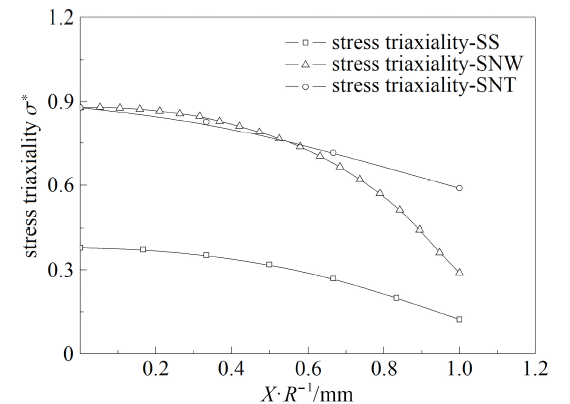Fig.20   Stress triaxiality on $X$ direction of SS, SNW and SNT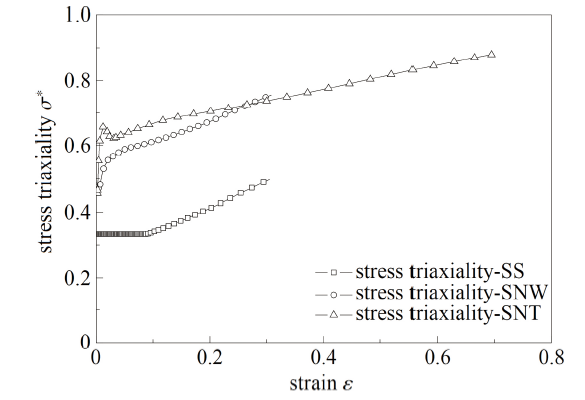Fig.21   The evolution of stress triaxiality on the center of the cross-section of SS, SNW and SNT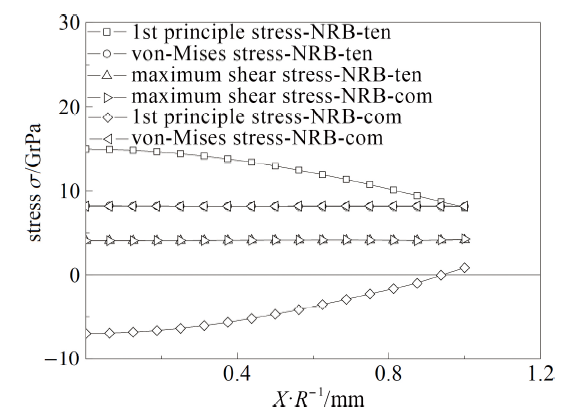Fig.22   Stress distribution on $X$ direction of NRB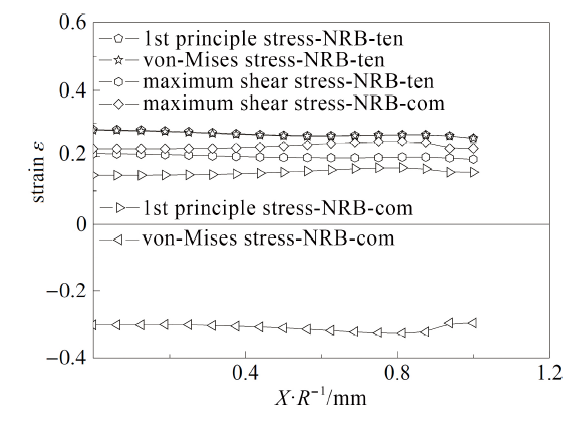Fig.23   Strain distribution on $X$ direction of NRB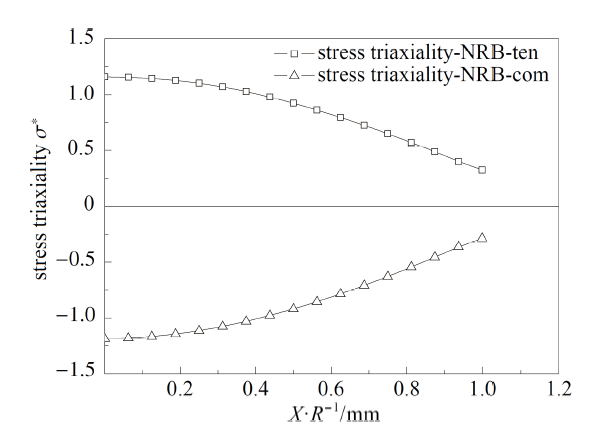Fig.24   Stress triaxiality on $X$ direction of NRB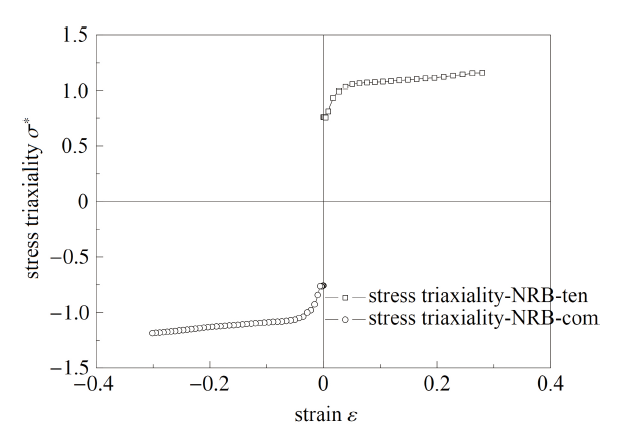Fig.25   The evolution of stress triaxiality on the center of the cross-section of NRB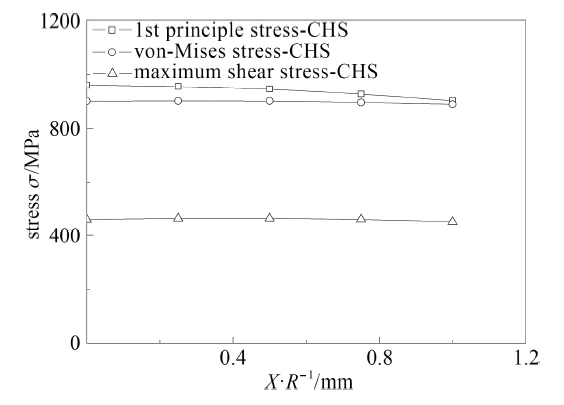Fig.26   Stress distribution on $X$ direction of CHS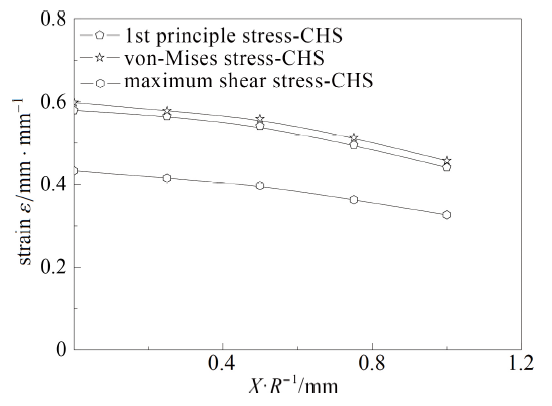Fig.27   Strain distribution on $X$ direction of CHS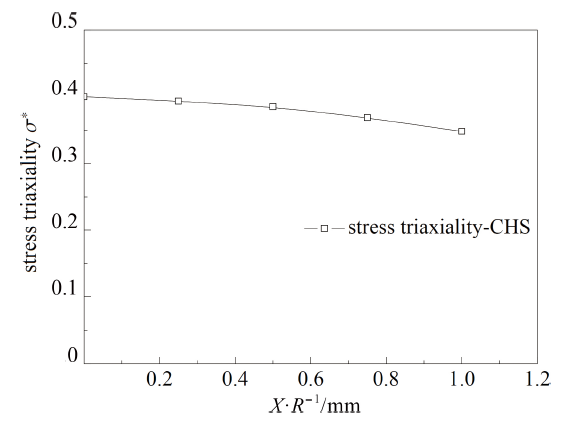Fig.28   Stress triaxiality on $X$ direction of CHS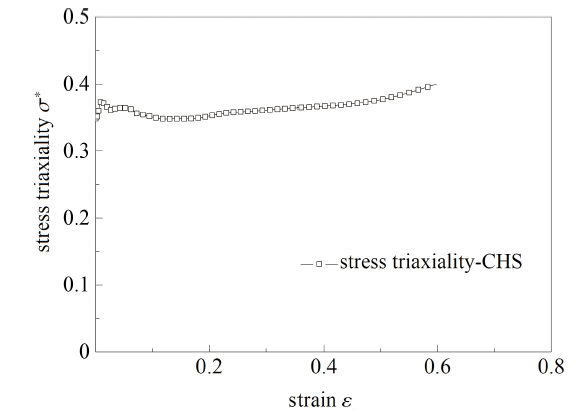Fig.29   The evolution of stress triaxiality on the center of the cross-section of CHS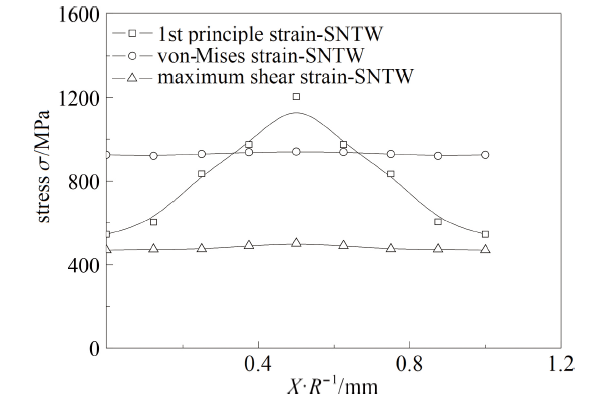Fig.30   Stress distribution on $X$ direction of SNTW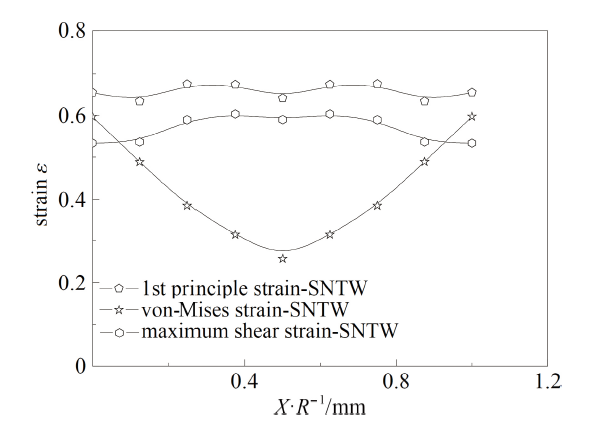Fig.31   Strain distribution on $X$ direction of SNTW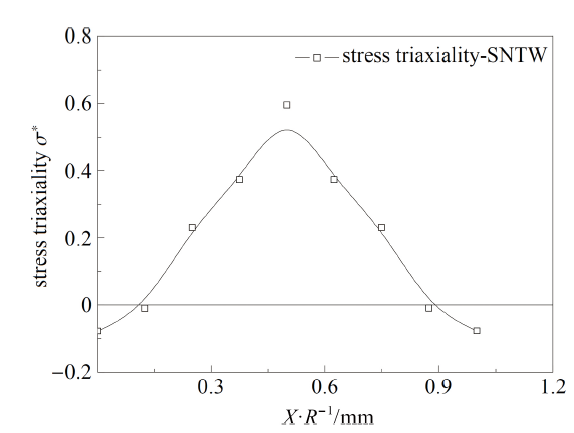Fig.32   Stress triaxiality on $X$ direction of SNTW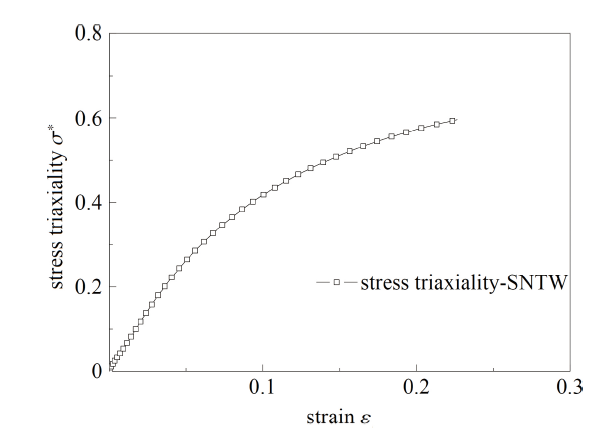Fig.33   The evolution of stress triaxiality on the center of the cross-section of SNTW

(1)从截面中心点到边缘, 第一主应力和应力三轴度分布趋势相同, 表现为逐渐降低, 而Mises等效应力和切应力变化不明显;

(2)对于拉伸试样和压缩试样, 第一主应变和Mises等效应变在数值上十分接近, SS, SNT和CHS试样的第一主应变、Mises等效应变和切应变从截面中心点到边缘逐渐降低, SNW试样和NRB试样的第一主应变、Mises等效应变和切应变变化不明显; 对于SNTW扭转试样, Mises 等效应变从中心点到边缘逐渐升高, 而第一主应变和切应变变化不明显;

(3)对于拉伸试样和压缩试样, 在试验初期, 随着试验的进行, 断裂面中心点的应变逐渐提高, 除SS和CHS试样外各试样当地应力三轴度变化较为剧烈, 在应变达到一定值以后逐渐缓慢增大直至破坏. SS和CHS试样临界断裂点在试验前半部分保持单轴应力状态, 应力三轴度约为0.33, 当SS试样应变达到0.1时, 应力三轴度逐渐升高直至破坏, CHS 试样直至断裂前才发生应力三轴度小幅度上升, 即在CHS试样的拉伸试样过程中, 试样的临界断裂点在大部分时间内保持单轴应力状态; 对于扭转试样, 因为其临界断裂点在试样边缘, 所以在试验过程中该材料单元的应力三轴度从0开始逐渐升高直至断裂.

### 3.2 材料临界断裂状态分析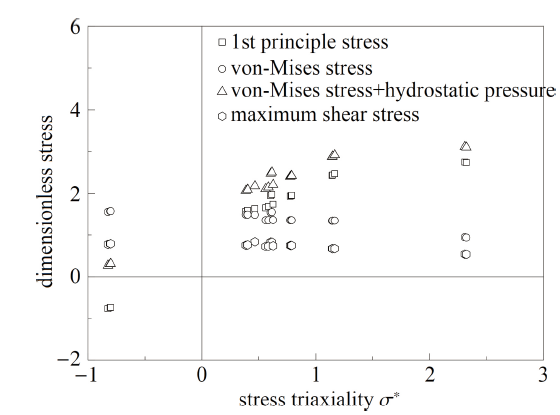Fig.34   The variations of different stress parameters with stress triaxiality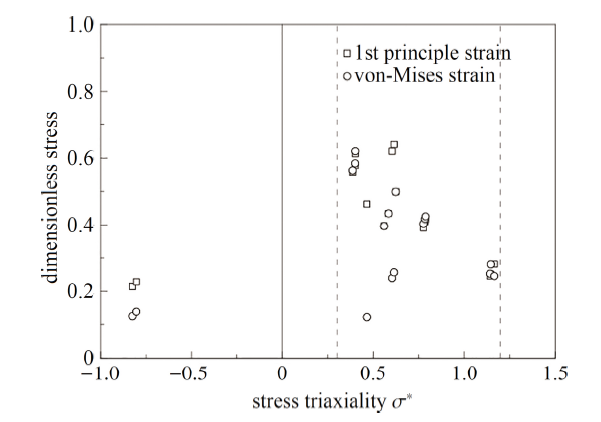Fig.35   The variations of different strain parameters with stress triaxiality

## 4 裂纹构型的启裂分析方法}

\begin{equation*}\left.\begin{split}&\dfrac{V_{\text P}}{V^*}=k_1\left(1-\dfrac{a}{W}\right)^{k_2}\\&\varepsilon_{\text{p-eqm}}=k_3\left(\dfrac{h_{\text p}}{h^*}\right)^{k_4}\end{split}\right\}\tag*{(6)}\end{equation*}

\begin{equation*}\left\lgroup\dfrac{PA_1}{V^*}\right\rgroup^N+\dfrac{PA_2}{V^*}=\dfrac{v}{v^*}\hskip 25mm\tag*{(7)}\end{equation*}\begin{equation*}\left.\begin{split}&A_1=\dfrac{(1+N)v^*}{(1+m_{\text p})NKk_1k_3^{1+1/N}\left(1-\dfrac{a}{W}\right)^{k_2}}\\&A_2=\dfrac{v^*}{k_5E\left(1-\dfrac{a}{W}\right)^{k_2}}\end{split}\right\}\tag*{(8)}\end{equation*}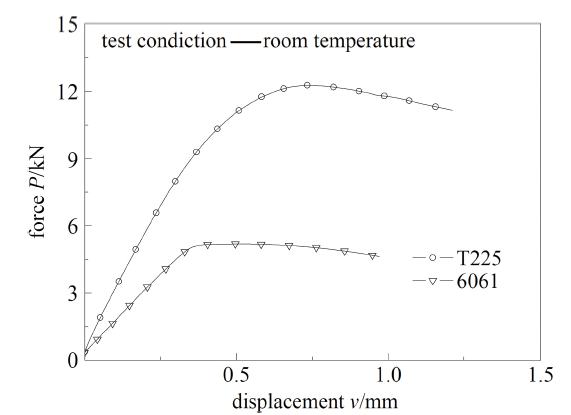Fig.36   The tensile $P$-$v$ curves of cracked specimens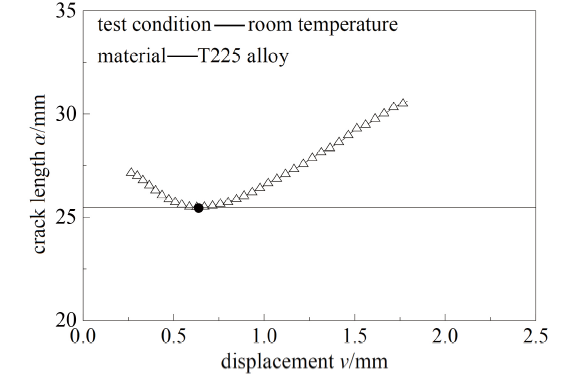Fig.37   The $a$-$v$ curve for SEB specimen of T225 alloy

## 5 临界延性断裂准则

\begin{equation*}\left(1+\dfrac{\sigma_\text {1f}}{\sigma_y}\right)=m_1(1+\sigma^*_\text{f})\text e^{ m_2(1+\sigma^*_\text f)}\tag*{(9)}\end{equation*}

\begin{equation*}1+\sigma^*=1+\dfrac{\sigma_\text m}{\sigma_\text{eq}}=\dfrac{\sigma_\text m+\sigma_\text{eq}}{\sigma_\text{eq}}\tag*{(10)}\end{equation*}

\begin{equation*}\int(1+\sigma^*)\text d\varepsilon=\dfrac{\int(\sigma_\text m+\sigma_\text{eq})\text d\varepsilon}{\int\sigma_\text{eq}\text d\varepsilon}\tag*{(11)}\end{equation*}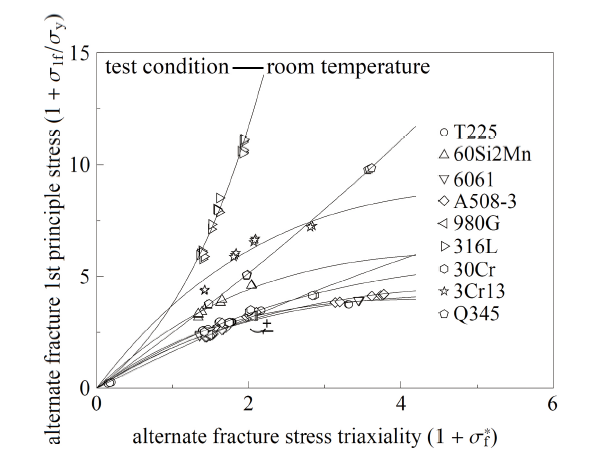Fig.38   The critical ductile fracture criterion of metal materials

Table 4   Parameters for unified critical fracture criterion of various materials

MatcrialM1m2
T2252.55-0.238
60Si2Mn3.31-0.202
60612.20-0.195
A508-32.14-0.173
980G1.71-0.043
316L2.410.442
30Cr2.23-0.145
3Cr134.49-0.187
Q3452.39-0.037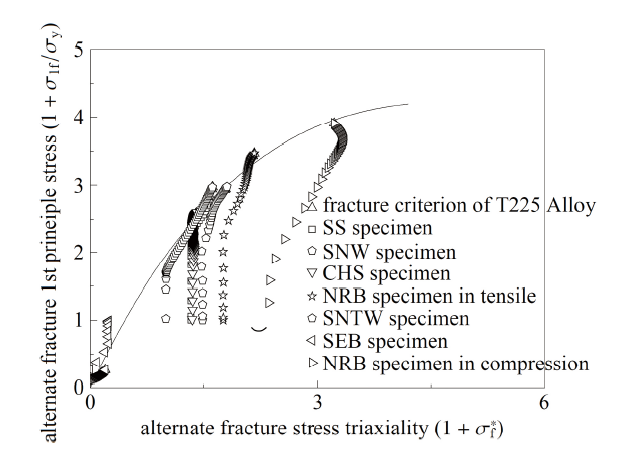Fig.39   Stress state points of T225 alloy specimens

Table 5   Critical values of fracture criterions in Refs.[1-5]

MaterialsFreudentalC-LR-TBrozzoOh
SS 1#3453710.7920.5620.559
SS2#2602640.6050.4310.430
SS3#2913020.6720.4780.476
SNW 1#3244290.8180.5670.548
SNW 2#3184200.8020.5560.538
SNW 3#3043990.7620.5300.512
CHS 1#5085230.8680.6300.604
CHS 2#4584700.7860.5710.545
CHS 3#5045180.8610.6240.599
NRB-ten-1#2133740.8300.4910.491
NRB-ten-2#1893290.7550.4370.448
NRB-ten-3#1843200.7620.4250.436
SNTW-1#5152740.4810.3280.350
SNTW-2#2071530.2800.1920.215
SNTW-3#7503160.5500.3730.396
NRB-com-1#1791090.088 60.2830.141
NRB-com-2#1811100.08930.2840.142
NRB-com-3#1661030.08260.2700.135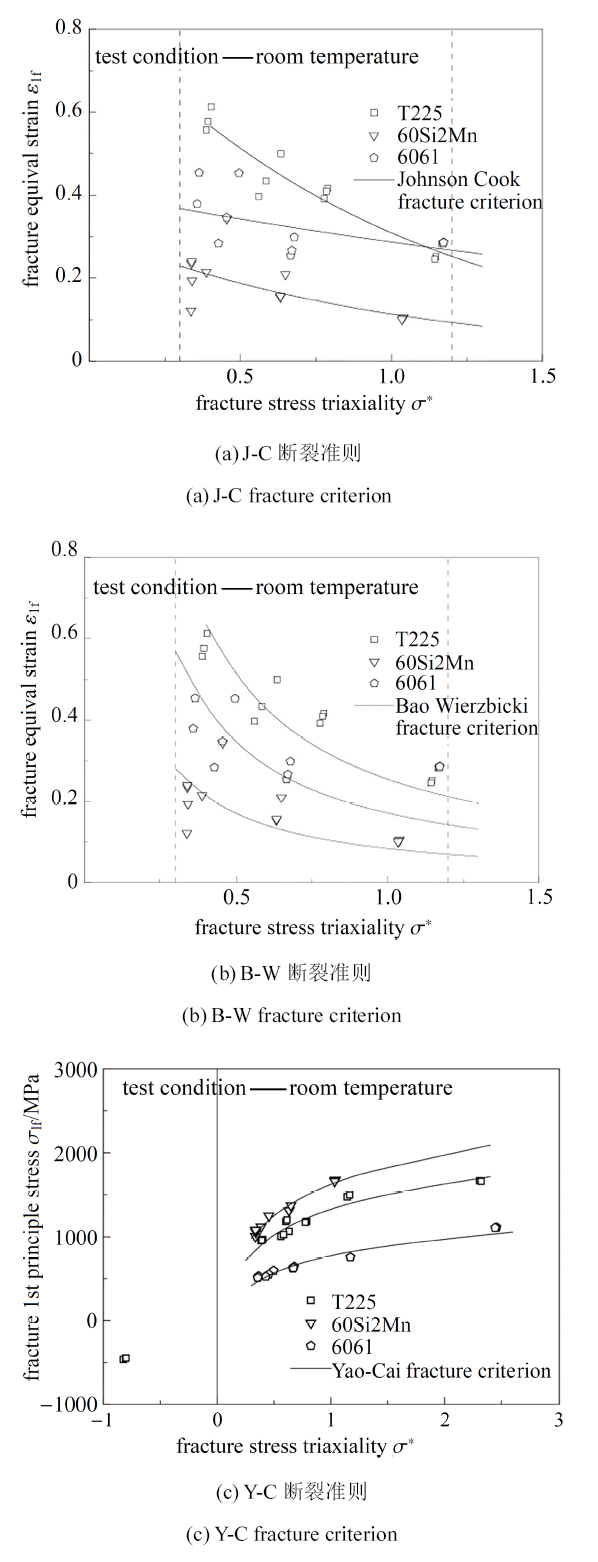Fig.40   The description of critical state for materials under different fracture criterions[22-23, 34]

J-C断裂准则和B-W断裂准则$(0.4\leqslant\sigma^*\leqslant0.95)$均以临界等效应变作为断裂阈值, 由图40图41可见, 对于3种材料, 在应力三轴度为$0.3\sim 1.2$的范围内, 大部分数据点集中在两种准则模型趋势线周围, 存在少量数据点距离趋势线较远, J-C断裂准则和B-W断裂准则对于描述延性材料的断裂行为均有一定的准确性, 但J-C 断裂准则预测结果明显优于B-W准则; Y-C断裂准则和本文提出的延性断裂准则均选取临界第一主应力作为断裂阈值, 由图42 可见, Y-C 断裂准则模型趋势线与试验数据点吻合良好, 可见依赖较窄三轴度范围的拉伸断裂试验而建立的Y-C 断裂准则对于较高三轴度的材料断裂也有良好适应性, 但对三轴度低于0.33的材料断裂结果缺乏良好描述的趋势.

## 6 结 论

(1)完成了9种金属材料具有不同约束程度的10类试样的延性断裂试验, 获得了拉、压、扭和裂尖断裂等破坏形式的材料载荷-位移试验关系.

(2)基于圆棒漏斗试样拉伸试验所得载荷-位移曲线, 采用FAT方法得到了9种材料直至破坏的全程等效应力- 应变关系, 以此作为材料本构通过有限元分析获得了10类试样直至破坏的载荷-位移关系, 模拟结果与试验结果有较好的一致性, 表明FAT方法所得各向同性材料试样颈缩后的等效全程本构关系具有真实性和普适性.

(3)基于全程材料等效本构关系曲线, 实现了对延性材料直至断裂的加载过程力学行为精确模拟, 对应9种金属材料、10类试样的36个载荷-位移临界断裂点, 获得了对应的材料临界断裂应力、应变与临界应力三轴度, 研究表明, 第一主应力在弹塑性变形过程中为控制断裂的主控参量.

(4)基于能量等效方法确定了裂纹构型的启裂点, 提出了高约束度条件下材料的断裂点应力状态的获取方法.

(5)通过研究光滑、缺口、裂纹构型的断裂状态, 提出了$-1$至3范围的应力三轴度下由第一主应力主控的统一临界延性断裂准则, 该准则表征了复杂应力状态下材料的约束程度和应力状态.

(6)获取了9种材料的临界延性断裂准则参数, 与已有延性断裂准则预测结果进行对比, 证明本文所提出延性断裂准则具有更好的准确性和普适性.

The authors have declared that no competing interests exist.

## 参考文献 原文顺序 文献年度倒序 文中引用次数倒序 被引期刊影响因子

  Freudenthal AM.The Inelastic Behaviour of Solids. New York: Wiley, 1950  Cockcroft MG, Latham DJ.Ductility and the workability of metals. J Inst Met., 1968, 96(1): 33-39  Rice JR, Tracey DM.On the ductile enlargement of voids in triaxial stress fields. Journal of the Mechanics and Physics of Solids, 1969, 17(3): 201-217  Brozzo P, Deluca B, Rendina R.A new method for the prediction of formability limits in metal sheets//Proc. 7th biennal Conf. IDDR. 1972  Oh SI, Kobayashi S.Workability of aluminum alloy 7075-T6 in upsetting and rolling. Journal of Engineering for Industry, 1976, 98(3): 800-806  Gurson AL.Continuum theory of ductile rupture by void nucleation and growth: Part I-Yield criteria and flow rules for porous ductile media. Journal of Engineering Materials and Technology, 1977, 99(1): 2-15  Tvergaard V.Influence of voids on shear band instabilities under plane strain conditions. International Journal of fracture, 1981, 17(4): 389-407  Tvergaard V.On localization in ductile materials containing spherical voids. International Journal of Fracture, 1982, 18(4): 237-252  Tvergaard V, Needleman A.Analysis of the cup-cone fracture in a round tensile bar. Acta Metallurgica, 1984, 32(1): 157-169  Needleman A, Tvergaard V.An analysis of ductile rupture in notched bars. Journal of the Mechanics and Physics of Solids, 1984, 32(6): 461-490  Nahshon K, Hutchinson JW.Modification of the Gurson model for shear failure. European Journal of Mechanics-A/Solids, 2008, 27(1): 1-17  Nielsen KL, Tvergaard V.Effect of a shear modified Gurson model on damage development in a FSW tensile specimen. International Journal of Solids and Structures, 2009, 46(3-4): 587-601  彭云强, 蔡力勋, 包陈等.基于材料全程本构关系对延性断裂韧性的数值模拟方法与应用. 工程力学, 2016, 33(5):11-17 (Peng Yunqing, Cai Lixun, Yao Di, et al.Numerical simulation methods and application for ductile fracture toughness based on full-range constitutive relationship. Engineering Mechanics, 2016, 33(5): 11-17 (in Chinese))  古斌, 郭宇立, 李群. 基于构型力断裂准则的裂纹与夹杂干涉问题. 力学学报, 2017, 49(6): 1312-1321 (Gu Bing, Guo Yuli, Li Qun.Crack interacting with an individual inclusion by the fracture criterion of configuration al force. Chinese Journal of Theoretical and Applied Mechanics, 2017, 49(6): 1322-1334 (in Chinese))  姚迪, 蔡力勋, 包陈等. 基于试验与有限元耦合技术的延性材料全程单轴本构关系获取方法. 固体力学学报, 2014, 35(3): 226-240 (Yao Di, Cai Lixun, Bao Chen, et al.Determination of stress-strain curve of ductile materials by testing and finite element coupling method. Chinese Journal of Solid Mechanics, 2014, 35(3): 226-240 (in Chinese))  蔡力勋, 姚迪, 包陈. 单轴拉伸全程真应力-真应变曲线测试技术.中国专利: 201210518207, 2013 (Cai Lixun, Yao Di, Bao Chen.The testing technology of materials tensile constitutive curves. Chinese Patent: 201210518207, 2013 (in Chinese))  Bai Y, Wierzbicki T.Application of extended Mohr-Coulomb criterion to ductile fracture. International Journal of Fracture, 2010, 161(1): 1  Mohr O.Abhandlungen aus dem gebiete der technischen mechanik. 1906  Chen X, Shi MF, Shih HC, et al.AHSS shear fracture predictions based on a recently developed fracture criterion. SAE International Journal of Materials and Manufacturing, 2010, 3(2010-01-0988): 723-731  Luo M, Dunand M, Mohr D.Experiments and modeling of anisotropic aluminum extrusions under multi-axial loading-Part II: Ductile fracture. Int. J. Plast International Journal of Plasticity, 2012, 32: 36-58  高江平, 杨华, 蒋宇飞等. 三剪应力统一强度理论研究. 力学学报, 2017, 49(6): 1322-1334 (Gao Jiangping, Yang Hua, Jiang Yufei, et al.Study of three-shear stress unified strength theory. Chinese Journal of Theoretical and Applied Mechanics, 2017, 49(6): 1322-1334 (in Chinese))  Johnson GR, Cook WH.Fracture characteristics of three metals subjected to various strains, strain rates, temperatures and pressures. Engineering Fracture Mechanics, 1985, 21(1): 31-48  Bao Y, Wierzbicki T.On fracture locus in the equivalent strain and stress triaxiality space. International Journal of Mechanical Sciences, 2004, 46(1): 81-98  Gentile D, Newaz G, Bonora N, et al.Ductile damage evolution under triaxial state of stress: Theory and experiments. Inter. J. Plast., 2005, 21(5): 981-1007  Bai Y, Wierzbicki T.A new model of metal plasticity and fracture with pressure and lode dependence. International Journal of Plasticity, 2008, 24: 1071  Hopperstad OS, Borvik T, Langseth M, et al.On the influence of stress triaxiality and strain rate on the behaviour of a structural steel. Part I. Experiments. European Journal of Mechanics-A/Solids, 2003, 22(1): 15-32  Anderson D, Winkler S, Bardelcik A, et al.Influence of stress triaxiality and strain rate on the failure behavior of a dual-phase DP780 steel. Materials & Design, 2014, 60(8): 198-207  Bridgman P W.Studies in Large Plastic Flow and Fracture. New York: McGraw-Hill, 1952  Eduardo E., Diego J.Celentano Experimental and numerical analysis of the tensile test using sheet specimens. Finite Elements in Analysis and Design, 2004, 40(5): 555-575  Zhang KS.Technical note fracture prediction and necking analysis. Engineering Fracture Mechanics, 1995, 52(3): 575-582  Brünig M.Numerical analysis and modeling of large deformation and necking behavior of tensile specimens. Finite Elements in Analysis and Design, 1998, 28(4): 303-319  Dumoulin S, Tabourot L, Chappuis C, et al.Determination of the equivalent stress-equivalent strain relationship of a copper sample under tensile loading. Journal of Materials Processing Technology, 2003, 133(1-2): 79-83  Joun MS, Eom JG, Lee MC.A new method for acquiring true stress-strain curves over a large range of strains using a tensile test and finite element method. Mechanics of Materials, 2008, 40(7): 586-593  Yao D, Cai L, Bao C.A new strength criterion for ductile materials based on a finite element aided testing method. Materials Science and Engineering: A, 2016, 673: 633-647  Chen H, Cai LX.Theoretical model for predicting uniaxial stress-strain relation by dual conical indentation based on equivalent energy principle. Materials Science and Engineering: A, 2016, 121: 181-189  Chen H, Cai L.Unified elastoplastic model based on a strain energy equivalence principle. Applied Mathematical Modelling, 2017, 52: 664-671  贺屹, 蔡力勋, 陈辉等. 求解I 型裂纹构元J 积分的半解析方法. 力学学报, 2018, 50(3): 579-588 (He Yi, Cai Lixun, Chen Hui, et al.A semi analytical method to solve J-integral for mode-I crack components. Chinese Journal of Theoretical and Applied Mechanics, 2018, 50(3): 579-588 (in Chinese))/

 〈〉